Visual Case Based Type Questions: Chemical Reactions & Equations - 1

# Visual Case Based Type Questions: Chemical Reactions & Equations - 1 - Science Class 10

Marble’s popularity began in ancient Rome and Greece, where white and off-white marble were used to construct a variety of structures, from handheld sculptures to massive pillars and buildings.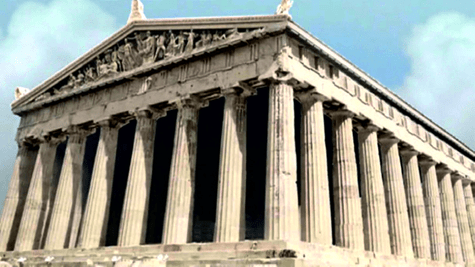Question 1:
The substance not likely to contain CaCO3 is
(a) Dolomite
(b) A marble statue
(c) Calcined gypsum
(d) Sea shells.

Let’s write the Chemical formula for all of them
Dolomite → (CaMg(CO3)2)
A marble statue → Recrystallized carbonate minerals commonly calcite (CaCO3) or dolomite (CaMg(CO3)2)
Calcined Gypsum →  CaSO4.2H2O;
Sea shells → CaCO3

Question 2:
Gas A, obtained above is a reactant for a very important biochemical process which occurs in the presence of sunlight. Identify the name of the process -
(a) Respiration
(b) Photosynthesis
(c) Transpiration
(d) Photolysis

The following reaction takes place when CaCO3 is heated;
CaCO3 → CaO + CO2
CO2 is the gas formed and it is utilized in the process of photosynthesis

Question 3:
Calcium oxide can be reduced to calcium, by heating with sodium metal. Which compound would act as an oxidizing agent in the above process?
(a) sodium
(b) sodium oxide
(c) calcium
(d) calcium oxide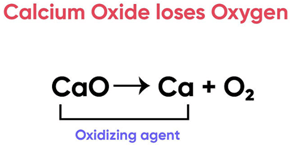Here, Calcium Oxide is losing Oxygen and undergoing reduction
A substance that undergoes reduction is an oxidising agent.
Thus Calcium Oxide is the oxidising agent

Chemistry in Automobiles:
For an internal combustion engine to move a vehicle down the road, it must convert the energy stored in the fuel into mechanical energy to drive the wheels. In your car, the distributor and battery provide this starting energy by creating an electrical "spark", which helps in combustion of fuels like gasoline. Below is the reaction depicting complete combustion of gasoline in full supply of air:
2C8H18(l) + 25O2(g) → 16 'X' + Y
Question 4:
Identify the types of chemical reaction occurring during the combustion of fuel:
(a) Oxidation & Endothermic reaction
(b) Decomposition & Exothermic reaction
(c) Oxidation & Exothermic reaction
(d) Combination & Endothermic reaction

Here
Decomposition Reaction:  The reaction in which complex compounds are broken down into simpler ones i.e. AB → A + B
Oxidation Reaction: The reaction in which an oxygen atom is gained i.e. A + O2 → AO2
Combination Reaction: When two or more substances combine to form a single product i.e. A + B → AB
And,
Endothermic Reaction: Endo means in and thermic means heat. In these reactions, heat is absorbed.
Exothermic Reaction: Exo means out and thermic means heat. In these reactions, heat is released.
Since in Combustion
Energy is released
Oxygen is gained
Thus, it is an oxidation and exothermic reaction
So, the correct answer is (c)

Question 5:
A student while walking on the road observed that a cloud of black smoke belched out from the exhaust stack of moving trucks on the road.’ Choose the correct reason for the production of black smoke:
(a) Limited supply of air leads to incomplete combustion of
(b) Rich supply of air leads to complete combustion of fuel.
(c) Rich supply of air leads to a combination reaction.
(d) Limited supply of air leads to complete combustion of fuel.

In the presence of limited supply of air, there isn’t enough oxygen to carry out complete combustion of fuels. Therefore, incomplete combustion takes place and a black sooty flame is observed Behind black smoke from car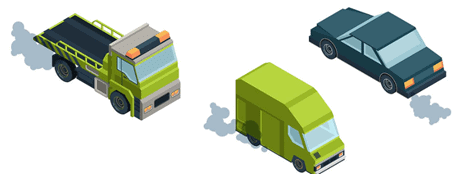The physical states of the reactants and products can be represented by using the symbols (s) for solids, (l) for liquids, (g) for gases and (aq) for aqueous solution along with their respective formulae. The word aqueous is written if the reactant or product is present as a solution in water. Precipitate can also be represented by using an arrow pointing downwards (↓) instead of using symbol (s).
In the same way, the gaseous state of an evolved gas can be represented by using an arrow pointing upward direction (↑) instead of using symbol (g). The specific condition of the reaction like temperature, pressure, catalyst etc. is written above or below the arrow in the chemical equation.

Question 6:
If the reactant or product is present as a solution of water, it is represented as:
(a) (s)
(b) (l)
(c) (aq)
(d)

Reaction of Silver Nitrate with Sodium Chloride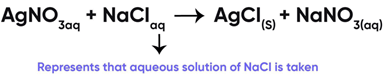Question 7:
Complete the missing variable given as X and Y in the following reaction:
2Na (s) + 2H2O (l) → 2NaOH (X) + H2 (Y)
(a) (aq) and (g)
(b) (s) and (g)
(c) (g) and  (l)
(d) (g) and (aq)

Here,
Since Na is reacting with water, the NaOH produced will be in aqueous medium, hence (aq) symbol will be used.
H2 is a gas which is evolved during the reaction, so we use the symbol (g) for it.
So, the correct answer is (a) (aq) and (g)

Question 8:
Which of the following reactions is correct?
(a)
Mg (aq) + H2SO4 (aq) → MgSO4 (aq) + H2
(b)
Mg (s) + H2SO4 (aq) → MgSO4 (aq) + H2
(c)
Mg (s) + H2SO4 (l) → MgSO4 (l) + H2(g)
(d)
Mg (s) + H2SO4 (l) → MgSO4 (s) + H2

A balanced chemical equation is one in which the number of atoms of both reactants and products are equal.
In reaction I: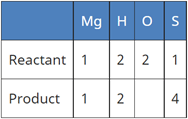In reaction II: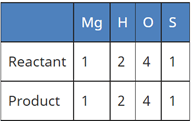Since the number of all atoms in reactants and products is equal, it is a balanced equation.
In reaction III: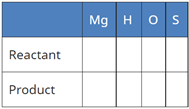In Reaction IV: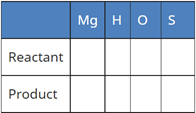In the following chemical reaction ‘‘zinc oxide reacts with carbon to produce zinc metal and carbon monoxide.’’ Answer any four question from I to V.
ZnO + C → Zn + CO

Question 9:
Name the substance getting oxidised and reduced in the above reaction:
(a) C and ZnO
(b) Zn and C
(c) ZnO and CO
(d) CO and  ZnO

Oxidation and Reduction Reaction Example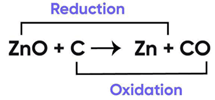Now,
Addition of oxygen is known as oxidation
Removal of oxygen is known as reduction.
Since in the equation
Oxygen is being removed from ZnO
Therefore, C is undergoing oxidation and ZnO reduction.
So, the correct answer is (a) C and ZnO

Question 10:
The reduction reaction involves:
(a)
gain of electrons
(b)
loss of electrons
(c)
increase in oxidation state
(d)

Reduction is the transfer of electrons between species in a chemical reaction where there is a process of gaining electrons or a decrease in the oxidation state by an element. A reduction chemical reaction involves increasing electrons associated with a single atom or a group of atoms.

Question 11:
The reactions used in black and white photography:
(i) Decomposition of silver bromide
(ii) Decomposition of silver chloride
(iii) Both
(iv) None of the above

Black and white photography is looks like
The photographic papers are prepared by using silver chloride and silver bromide which are initially white in color.
On exposure to air, AgCl and AgBr decompose and lose their color and black and white images are obtained.
So, the correct answer is (c) Both
Reactions Involved In Black And White Photography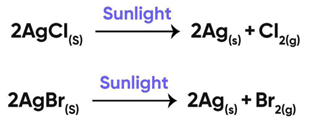P, Q and R are 3 elements which undergo chemical reactions according to the following equations Answer any four question from I to V:
(i) P2O3 + 2Q → Q2O3 + 2P
(ii) 3RSO4 + 2Q → Q2(SO4)3 + 3R
(iii) 3RO + 2P → P2O3 + 3R
Question 12:
The most reactive and the least reactive elements are:
(a) Q and P
(b) Q and R
(c) R and Q
(d) R and P

In the first reaction , Q is able to replace P from its salt i.e. P2 O3, Q is more reactive than P.
In the second reaction, Q is also able to replace R from its salt solution i.e. RSO4, Q is more reactive than R.
In the third reaction, P is able to replace r from its oxide, so, P is more reactive than R
Therefore, the r eactivity order is: Q > P > R (Q being the most reactive and R being the least)
Thus,
Most Reactive is Q
Least Reactive is R
So, the correct answer is (b)

Question 13:
3RSO4 + 2Q → Q2(SO4)3 + 3R
The given reaction shows:
(a) Q is more reactive than R
(b) Q is less reactive than R
(c) Q and R are equally reactive
(d) none of the above

Since in this reaction, Q is able to replace R from its salt RSO 4, Therefore, Q is more reactive than R.
So, the correct answer is (a)

Question 14:
Na2SO4 (aq) + BaCl2(aq) → BaSO4 (s) + 2NaCl(aq)
The above reaction is an example of:
(a) Double displacement reaction.
(b) Displacement reaction.
(c) Can be both.
(d) None of the above.

Since in this reaction the two reacting compounds are switching their positive and negative ions, it is a double displacement reaction.
So, the correct answer is (a)

Question 15:
The type of reaction is:
(a) Displacement reaction
(b) Combination reaction
(c) Neutralisation reaction
(d) Substitution reaction

Let’s look at all the options
Displacement Reaction: Reaction in which more reactive element takes the place of less reactive element.
Combination Reaction: When two or more substances react to form a single product, it is known as combination reaction.
Neutralisation reaction: A reaction in which acid and base react to form salt and water
Substitution Reaction: In this reaction, one functional group in a chemical compound is replaced by another.
Since in the above mentioned reactions, the more reactive elements (Q, P) are replacing the less reactive element (R), this is a displacement reaction.
So, the correct answer is (a) Displacement reaction

The document Visual Case Based Type Questions: Chemical Reactions & Equations - 1 | Science Class 10 is a part of the Class 10 Course Science Class 10.
All you need of Class 10 at this link: Class 10

## Science Class 10

78 videos|509 docs|153 tests

## FAQs on Visual Case Based Type Questions: Chemical Reactions & Equations - 1 - Science Class 10

 1. What is a chemical reaction?Ans. A chemical reaction is a process in which one or more substances undergo a chemical change to form new substances with different properties.
 2. How are chemical reactions represented?Ans. Chemical reactions are represented using chemical equations, where the reactants are written on the left side and the products are written on the right side, separated by an arrow.
 3. What is the law of conservation of mass in chemical reactions?Ans. The law of conservation of mass states that the total mass of the reactants in a chemical reaction is equal to the total mass of the products. This means that mass is neither created nor destroyed during a chemical reaction.
 4. How can you balance a chemical equation?Ans. To balance a chemical equation, you need to adjust the coefficients (numbers in front of the chemical formulas) so that the number of atoms of each element is the same on both sides of the equation. This is done by using trial and error or following specific rules.
 5. What are the different types of chemical reactions?Ans. There are several types of chemical reactions, including combination reactions, decomposition reactions, displacement reactions, double displacement reactions, and combustion reactions. Each type involves different changes and interactions between the reactants and products.

## Science Class 10

78 videos|509 docs|153 testsExplore Courses for Class 10 examSignup to see your scores go up within 7 days! Learn & Practice with 1000+ FREE Notes, Videos & Tests.
10M+ students study on EduRev
Track your progress, build streaks, highlight & save important lessons and more!
Related Searches

,

,

,

,

,

,

,

,

,

,

,

,

,

,

,

,

,

,

,

,

,

;